#### You may also like### Calendar Capers

Choose any three by three square of dates on a calendar page...Make a set of numbers that use all the digits from 1 to 9, once and once only. Add them up. The result is divisible by 9. Add each of the digits in the new number. What is their sum? Now try some other possibilities for yourself!### Doodles

Draw a 'doodle' - a closed intersecting curve drawn without taking pencil from paper. What can you prove about the intersections?

##### Age 11 to 16Challenge Level

If you prefer to work on paper, you may wish to print out some sheets of circles:
9 dot 10 dot 12 dot 15 dot 18 dot

In the GeoGebra interactivity below there is a circle with 9 equally spaced points on the edge, and one in the centre.

Draw as many different triangles as you can, by joining the centre dot and any two of the dots on the edge.

Can you work out the angles in your triangles?

You should have found four different triangles with angles of:
40, 70, 70
80, 50, 50
120, 30, 30,
160, 10, 10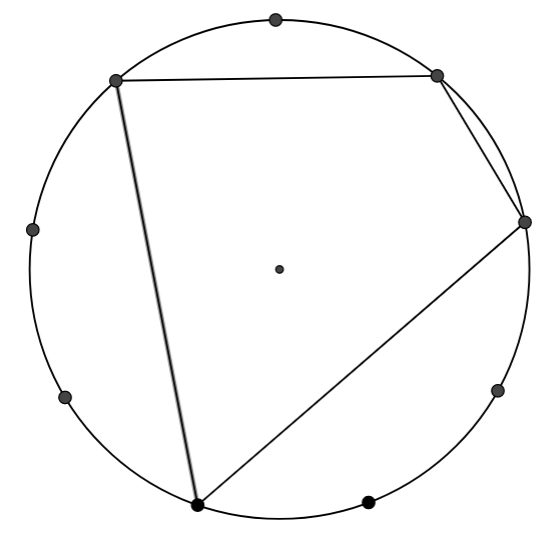Now draw a few quadrilaterals whose interior contains the centre of the circle, by joining four dots on the edge.

If you're finding it hard to work out the angles, take a look at Getting Started.

Create at least five different quadrilaterals in this way and work out their angles.

Perhaps you are wondering whether this only happens with 9-dot circles...

You may wish to explore the opposite angles of quadrilaterals on circles with a different number of dots.

Click below for interactivities with 10, 12, 15 and 18 dots around the circle.

10 dots

12 dots

15 dots

18 dots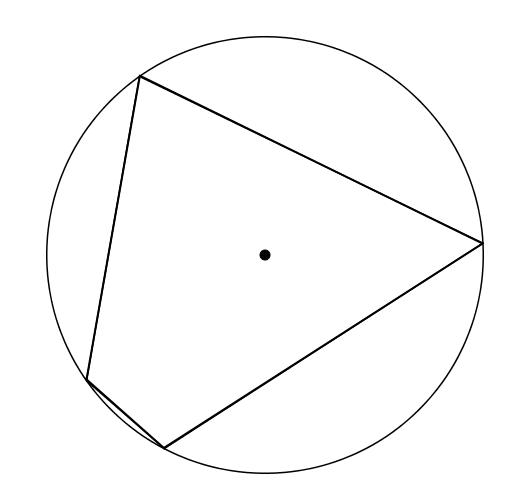Charlie drew a circle and chose four points at random to form a quadrilateral.

Can you prove that the opposite angles of his quadrilateral also add to $180^\circ$?

Click below to see a diagram that might help you to prove it.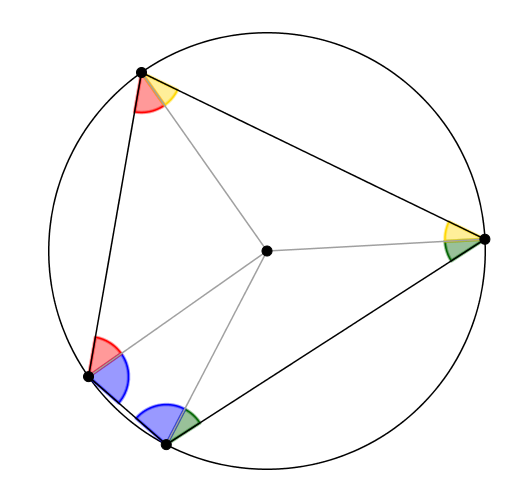Quadrilaterals whose vertices lie on the edge of a circle are called Cyclic Quadrilaterals.

So far you have only considered what happens to the opposite angles of cyclic quadrilaterals which contain the centre of the circle inside them...

Extension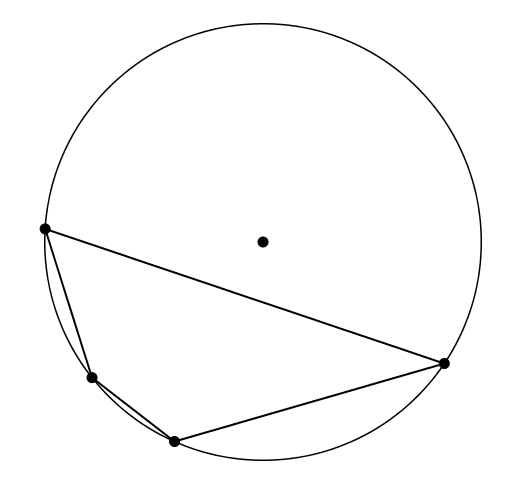Can you prove that when the centre of the circle is outside the cyclic quadrilateral, the opposite angles also add to $180^\circ$?

You may wish to draw some examples on 9, 10, 12, 15 and 18 dot circles.

Click below to see a diagram that might help you to prove that the opposite angles of cyclic quadrilaterals add to $180^\circ$.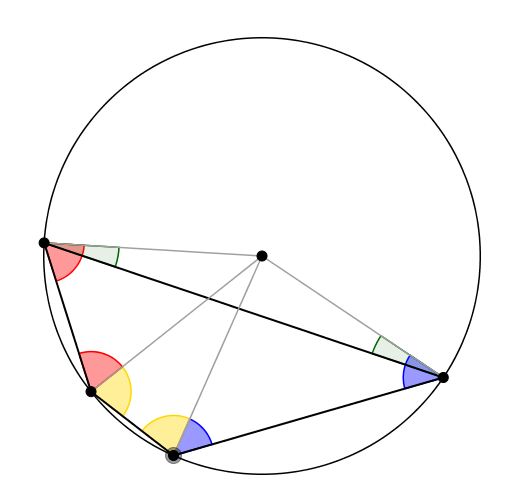You may be interested in the other problems in our Sharpen your Skills Feature.